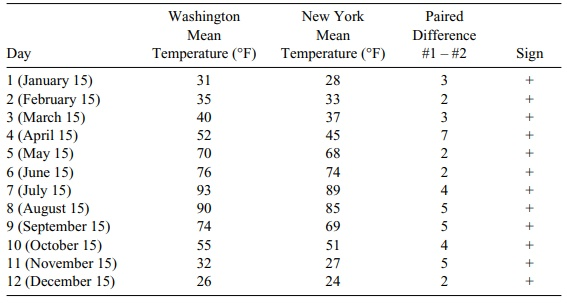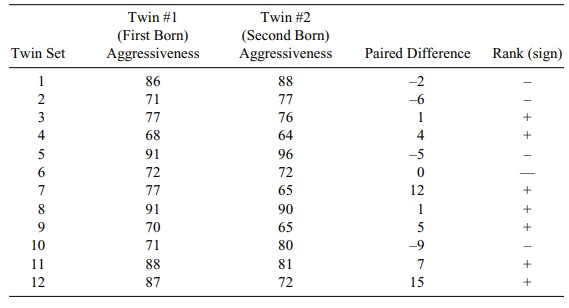# Sign Test

| Home | | Advanced Mathematics |

## Chapter: Biostatistics for the Health Sciences: Nonparametric Methods

The sign test is very much like the signed-rank test, only simpler.

SIGN TEST

The sign test is very much like the signed-rank test, only simpler. Again we com-pute the paired differences, but instead of determining the ranks of the absolute dif-ferences we just keep track of the number of positive (or negative differences). The sign of paired differences will have a binomial distribution with parameter p. If we define p (the binomial success parameter) to be the probability of a positive sign, and we eliminate cases with zero for the paired difference, then the parameter p will be equal to 0.5 under the null hypothesis. So the sign test is simply a test that a bi-nomial parameter p = 0.5, versus either a one-sided or two-sided alternative. Let us look at the two examples from the previous section to illustrate the sign test. First we will consider the temperature data for the two cities and then the example of ag-gressiveness among twins.

Referring to Table 14.6, we see that the number of successes is 12, meaning that for every month the temperature was higher in Washington than in New York. The p-value for the test is defined as the probability of as extreme or a more extreme outcome than the observed one under the null hypothesis. We see that the p-value is (1/2)12 = 0.000244. Remember from Chapter 5 that this probability is equivalent to the probability of 12 consecutive heads in a coin toss experiment with a fair coin. From this information, we can see that the significance of the test is less than p = 0.05 or p = 0.001, indicating that the differences are highly significant. In general, the sign test is not as powerful as the signed-rank test because it disregards the in-formation in the rank of the difference. Yet, in Table 14.6, the evidence is very strong that the p-value is small, even for the sign test. Now let us apply the sign test to the twin data (Table 14.7).

TABLE 14.6. Daily Temperatures for Two CitiesIn this case, the p-value is the probability of getting 7 or more successes (shown in Table 14.7 as 7 positive differences) in 11 trials when the binomial probability of success is p = 0.50. The probability of observing 7 or more successes in 11 trials when p = 0.50 is found to be 0.2744. So a p-value of 0.2744 indicates that the ob-served number of successes easily could have happened by chance. Therefore, we cannot reject the null hypothesis.

TABLE 14.7. Aggressiveness Scores for 12 Sets of Identical Twins